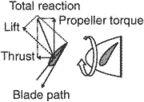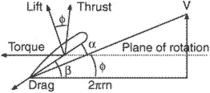# torque

(redirected from torqued)
Also found in: Dictionary, Thesaurus, Medical, Idioms, Wikipedia.

## torque,

in physics, that which tends to change the rate of rotation of a body; also called the momentmoment,
in physics and engineering, term designating the product of a quantity and a distance (or some power of the distance) to some point associated with that quantity.
of forceforce,
commonly, a "push" or "pull," more properly defined in physics as a quantity that changes the motion, size, or shape of a body. Force is a vector quantity, having both magnitude and direction.
. The torque produced by rotating parts of an electric motor or internal-combustion engine is often used as a measure of its ability to do useful work. The magnitude of the torque acting on a body is equal to the product of the force acting on the body and the distance from its point of application to the axis around which the body is free to rotate. Only the component of the force lying in the plane of rotation and perpendicular to the radius from the axis of rotation to the point of application contributes to the torque. This radius is called the moment arm, or lever arm. The net torque acting on a body is always equal to the product of the body's moment of inertia about its axis of rotation and its observed angular accelerationacceleration,
change in the velocity of a body with respect to time. Since velocity is a vector quantity, involving both magnitude and direction, acceleration is also a vector. In order to produce an acceleration, a force must be applied to the body.
. If a body undergoes no angular acceleration, there is no net torque acting on it. Units of torque are units of force multiplied by units of distance, e.g., newton-meters, dyne-centimeters, and foot-pounds (or pound-feet).

## Torque

The product of a force and its perpendicular distance to a point of turning; also called the moment of the force. Torque produces torsion and tends to produce rotation. Torque arises from a force or forces acting tangentially to a cylinder or from any force or force system acting about a point. A couple, consisting of two equal, parallel, and oppositely directed forces, produces a torque or moment about the central point. A prime mover such as a turbine exerts a twisting effort on its output shaft, measured as torque. In structures, torque appears as the sum of moments of torsional shear forces acting on a transverse section of a shaft or beam. See Couple

McGraw-Hill Concise Encyclopedia of Physics. © 2002 by The McGraw-Hill Companies, Inc.

## torque

[tȯrk]
(mechanics)
For a single force, the cross product of a vector from some reference point to the point of application of the force with the force itself. Also known as moment of force; rotation moment.
For several forces, the vector sum of the torques (first definition) associated with each of the forces.
McGraw-Hill Dictionary of Scientific & Technical Terms, 6E, Copyright © 2003 by The McGraw-Hill Companies, Inc.

## torque

That which tends to produce rotation; the product of a force and a lever arm which tends to twist a body, as the action of a wrench turning a nut on a bolt.
McGraw-Hill Dictionary of Architecture and Construction. Copyright © 2003 by McGraw-Hill Companies, Inc.

## torqueRotation of propeller produces torque.The illustration shows torque of a propeller. Here 2πrn is the rotational velocity. The direction of the torque is opposite to that of the direction of rotation of propeller.
The moment of force or combination of forces that tends to produce rotational motion. Torque is the counterforce against the engine force that drives a propeller or rotor. It is measured by multiplying the force by its perpendicular distance from the turning point.